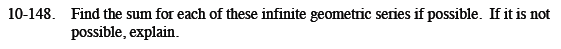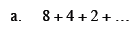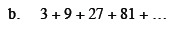Home > CCA2 > Chapter 10 > Lesson 10.3.1 > Problem10-148

10-148.
1. Find the sum for each of these infinite geometric series if possible. If it is not possible, explain. Homework Help ✎

1. 8 + 4 + 2 + ...

2. 3 + 9 + 27 + 81 + ...Find the common ratio.

$\frac{4}{8}=\frac{1}{2}$

$\frac{2}{4}=\frac{1}{2}$

Use the equation for an infinite geometric series:

$\sum_{n=1}^{\infty}{ar^{n-1}}=\frac{a}{1-r}$

16See part (a).

Not possible. r > 1, and the terms keep increasing.